# Binomial Theorem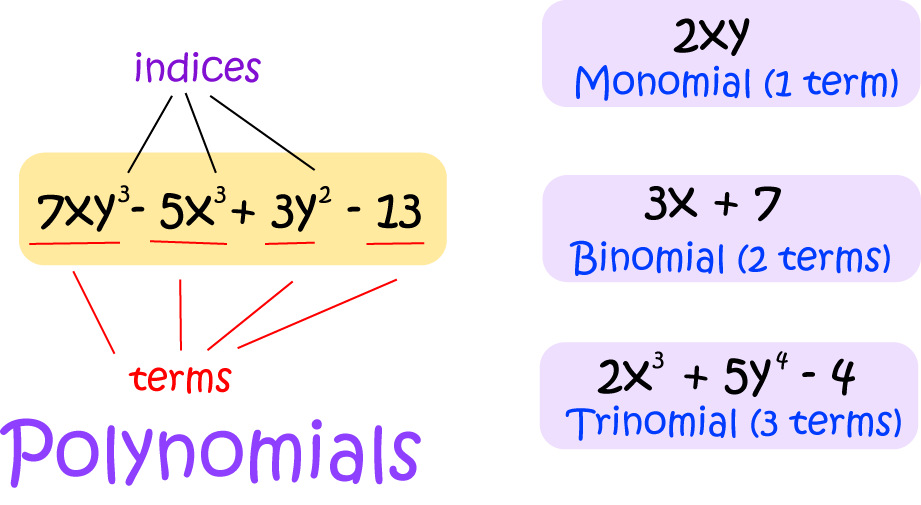A binomial is a polynomial with two terms. The Binomial Theorem tells us how to raise binomials to powers.

For example, $(a + b)$ is a binomial. Below are the powers of $(a+b)$ from $(a+b)^0$ up to $(a + b)^4$: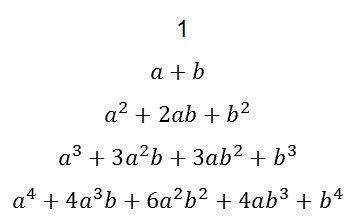and the coefficients are shown in green in the image below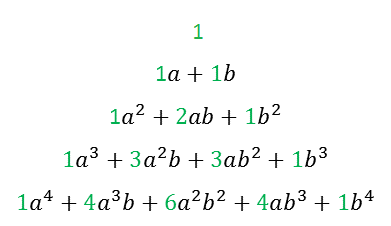We can feasibly calculate all of these powers using algebra, but the calculations just get longer and more tedious as we go along. Fortunately, the Binomial Theorem comes to the rescue. The Binomial theorem tells us that these coefficients are found on Pascal's Triangle. In fact, the green numbers shown in the image above form the first 5 rows of Pascal's Triangle: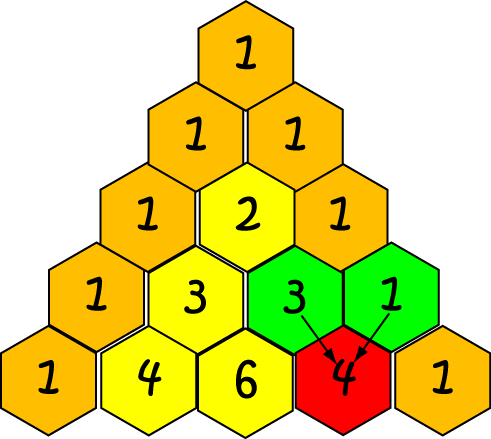We can easily build Pascal's triangle using the following steps.

We start at row zero, by placing a one on the top. Next, we add row one, which consists of two ones. We are then able to build up each additional row of the triangle as follows:

• Place 1s as the outside entries of the row.
• To obtain each of the interior entries, add the two entries of the triangle diagonally above it.
Repeat these steps to obtain as many rows of the triangle as you need.

In the diagram above, I show how to find one of the entries of the fifth row of the triangle. The entry, 4, coloured in red, is obtained by adding the two entries diagonally above it (coloured in green): $4 = 3 + 1$. We can similarly obtain the entry $6 = 3 + 3$ and the entry $4 = 1 + 3$ to complete the row.

Now, Pascal's triangle tells us the coefficients, but how do we find the powers of $a$ and $b$?

There are simple rules to follow. To find the terms of $(a + b)^n$, remember that

• The first term is $a^n$.
• The powers of $a$ go down by $1$ as we move from left to right.
• The powers of $b$ go up by $1$ as we move from left to right.
• The powers of $a$ and $b$ have to add up to $n$ (don't forget that $a^0 = 1$.
• The final term is $b^n$.
• The coefficients come from Pascal's triangle

Now let's look at a few more rows of Pascal's triangle: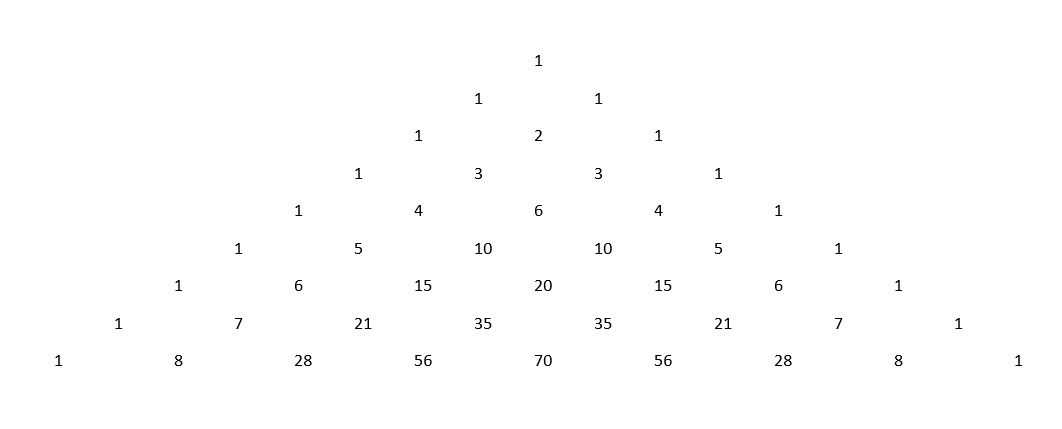and use them to find the exansion of $(a + b)^6$. Remember the rules above.
• The powers of $a$ are: $a^6 + a^5 + a^4 + a^3 + a^2 + a + 1$
• The powers of $b$ are: $1 + b + b^2 + b^3 + b^4 + b^5 + b^6$
• The coefficients are $1, 6, 15, 20, 15, 6, 1$, so the expansion is: $a^6 + 6a^5b + 15a^4b^2 + 20a^3b^3 + 15a^2b^4 + 6ab^5 + b^6$

That wasn't too bad, was it? It beats writing out all those products.

However, what if someone asked you for a term of the expansion of $(a + b)^{73}$? You really wouldn't want to write down the first $74$ rows of Pascal's triangle. Fortunately, there's an algebraic form of the binomial theorem that comes to the rescue. It gives us a formula for finding any coefficient we want without having to calculate all the coefficients that went before. Before we state it, let's start by finding a formula for the entries in Pascal's triangle.

## Finding Any Entry of the Triangle

The entries of Pascal's Triangle are actually combinations. Do you remember the definition?

$\begin{pmatrix} n \\ k\end{pmatrix} = \dfrac{n!}{k! (n - k)!}$
If you can't remember what $n!$ is, it's the product of all the integers less than or equal to $n$, all the way down to $1$. For example,
• $3! = 3 \times 2 \times 1$
• $7! = 7 \times 6 \times 5 \times 4 \times 3 \times 2 \times 1$

Here's part of the triangle, with entries written as combinations:And now you can see why we call the first row and the first column row and column zero.

#### Example

What is the entry in row 6 and term 3 (remember the rows and columns are numbered, starting at 0) of Pascal's triangle?

Solution:

The entry is $\begin{pmatrix} 6 \\ 3 \end{pmatrix} = \dfrac{6!}{3! (6 - 3)!} = \dfrac{6!}{3!3!} = \dfrac{6 \times 5 \times 4}{3 \times 2 \times 1} = 20$ and that matches the entry in the triangle. So, it works. You should probably try a few more for yourself.

Now, to get a single term of a binomial expansion, we need to remember that in $(a + b)^n$, the powers have to add to $n$, the powers of $a$ go down and the powers of $b$ go up. The coefficient of the term including $b^k$ is $\begin{pmatrix}n\\k \end{pmatrix}$, so the term is:

$\begin{pmatrix} n \\ k \end{pmatrix} a^{n - k}b^k$

It can be very useful to be able to calculate a single term of the binomial expansion.

## Summation Notation

Before I can state the algebraic form of the binomial theorem, I need to talk about summation notation. This is just notation that lets you write long sums in a shorthand way. Here's an example: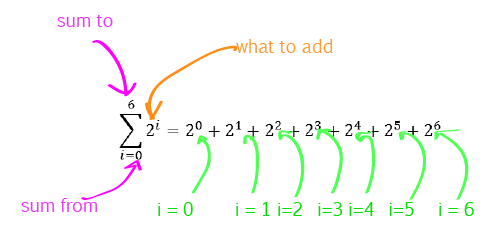## The Algebraic Form of the Binomial Theorem

Now we know what all the parts of this formula mean, we can write it down:

$(a + b)^n = \sum_{k=0}^n \begin{pmatrix} n \\ k \end{pmatrix} a^{n - k}b^k$

Let's use it in anger on an example.

### Example

Write down the expansion of $(a + b)^4$:

\begin{align*} (a + b)^4 &= \sum_{k=0}^4 \begin{pmatrix}4 \\k\end{pmatrix} a^{4 - k}b^k\\ &= \begin{pmatrix} 4\\ 0\end{pmatrix} a^4 + \begin{pmatrix} 4\\ 1\end{pmatrix} a^3b + \begin{pmatrix} 4\\ 2\end{pmatrix} a^2 b^2 + \begin{pmatrix} 4\\ 3\end{pmatrix} ab^3 + \begin{pmatrix} 4\\ 4\end{pmatrix} b^4\\ &= 1 (a^4) + 4(a^3b) + 6 (a^2 b^2) + 4(ab^3) + 1(b^4)\\ &= a^4 + 4a^3b + 6a^2b^2 + 4ab^3 + b^4 \end{align*}

The theorem is really only useful for larger values of $n$. For smaller values, it is usually easier to remember the rules:

• The powers of $a$ go down
• The powers of $b$ go up
• The powers of $a$ and $b$ add to $n$
• The coefficients come from Pascal's triangle.

Sometimes we need to find just one term from a binomial expansion. Let's look at a couple of examples.

### Example

Find the coefficient of $x^4$ in the expansion of $(3x + 2)^7$.

Solution:

$x^4$ appears in the term in $(3x)^4(2)^3 = 81x^4 (8) = 648x^4$.

The coefficient of this term is $\begin{pmatrix}7\\3\end{pmatrix} = \dfrac{7!}{4!3!} = \dfrac{7 \times 6 \times 5}{3 \times 2 \times 1} = 35$ (or we can read it from Pascal's triangle)

So, the term is $35 \times 648 x^4 = 22,680x^4$, and its coefficient is $22,680$.

### Example

Find the term that does not include $x$ in the expansion of $\left(x + \dfrac{1}{x}\right)^6$.

Solution:

The term in $x^3 \left(\dfrac{1}{x}\right)^3$ does not include $x$.

Its coefficient is $\begin{pmatrix}6\\3\end{pmatrix} = \dfrac{6!}{3!3!} = \dfrac{6 \times 5 \times 4}{3 \times 2 \times 1} = 30$ (or we can read it from Pascal's triangle).

So, the term that does not contain $x$ is $30$.

## Some Applications of the Binomial Theorem

In the article on Pascal's triangle, we said that the rows of Pascal's triangle all add up to powers of two. Here's the reason why: each row corresponds to a different power of $(1 + 1) = 2$, so its entries will add up to $2^n$ for some $n$.

This is an advanced example: Euler's number $e = 2.71828\dots$ can be written as the limit of binomial expansions. As $n$ gets very large, the binomial expansion $\left(1 + \dfrac{1}{n}\right)^n$ approaches $e$.

We can use the binomial theorem to calculate probabilities for experiments that only have two possible outcomes. We'll talk about that some more in the article on binomial probability.

### Description

This chapter series is for Year 10 or higher students, topics include

• Arranging Objects in Lines
• Factorial
• Subsets
• Four colour theorem

and more

### Audience

Year 10 or higher students

### Learning Objectives

These chapters are related to data and in particular "Counting" topics such as Binomial Theorem, Subsets etc

Author: Subject Coach
You must be logged in as Student to ask a Question.